# Trajectory Formula

Trajectory Formula

A projectile is an object that is given an initial velocity, and is acted on by gravity. The path the object follows is called its trajectory. The trajectory has horizontal (x) and vertical (y) position components. If a projectile is launched with an initial velocity v0, at an angle θ from the horizontal plane, then its vertical position can be found from its horizontal position using the following formula. The units of horizontal and vertical position are meters (m).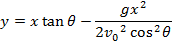y = vertical position (m)

x = horizontal position (m)

v0 = initial velocity (combined components, m/s)

g = acceleration due to gravity (9.80 m/s2)

θ = angle of the initial velocity from the horizontal plane (radians or degrees)

Trajectory Formula Questions:

1) A baseball player hits a ball, sending it away from the bat at a velocity of 45.0 m/s, and an angle of 66.4° relative to the field. In the ball's direction of travel, the end of the field is 140.0 m away. What is the height of the ball when it reaches the end of the field?

Answer: The height of the ball is its vertical position. The horizontal position of interest in this question is the end of the field, at x = 140.0 m. The vertical position y must be solved for. The angle θ and initial velocity v0 are presented in the question. The trajectory formula can be used to solve for y: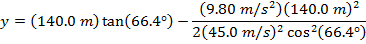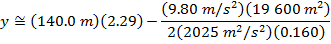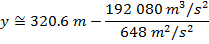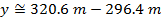The vertical position of the ball at the end of the field (x = 140.0 m) is.

2) A water skier has set up a stunt in which he plans to jump over a burning obstacle. The fire will be 4.00 m away from the ramp, and 1.00 m taller than the height of the ramp. If the ramp is inclined at an angle of 36.9° relative to the water, and he plans to take off from the ramp at a velocity of 9.00 m/s, will he be able to jump over the flames?

Answer: The horizontal position of interest is the position of the burning obstacle, relative to the ramp. This means that the x position is x = 4.00 m. The vertical position y must be solved for. The angle θ and initial velocity v0 are presented in the question. The trajectory formula can be used to solve for y: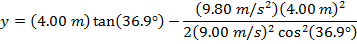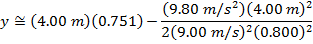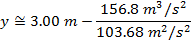The vertical position of the skier at a horizontal position of x = 4.00 m will be. This is more than the 1.00 m height of the burning obstacle, so the water skier will be able to jump over it.